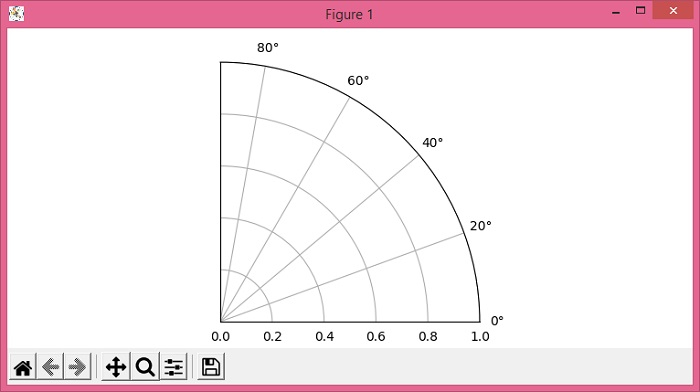# How to plot half or quarter polar plots in Matplotlib?

To plot half or quarter polar plots in Matplotlib, we can take the following steps −

• Set the figure size and adjust the padding between and around the subplots.

• Create a new figure or activate an existing figure using figure() method.

• Add an axes to the figure as part of a subplot arrangement.

• For half or quarter polar plots, use set_thetamax() method.

• To display the figure, use show() method.

## Example

from matplotlib import pyplot as plt

plt.rcParams["figure.figsize"] = [7.50, 3.50]
plt.rcParams["figure.autolayout"] = True

fig = plt.figure()
plt.show()